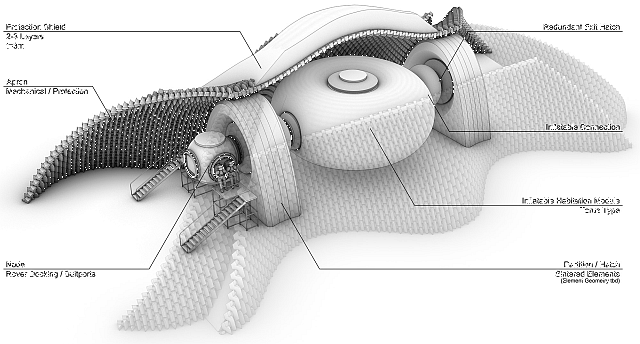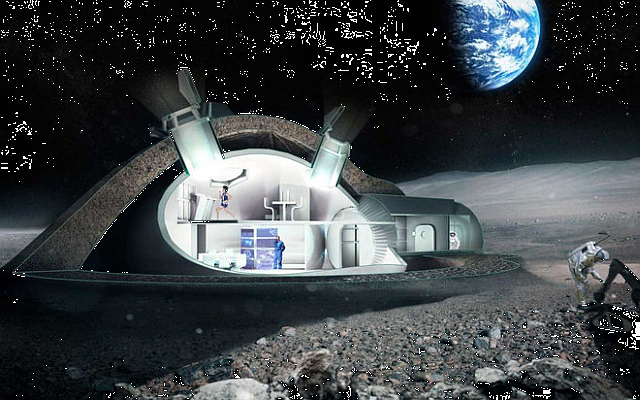# Radiation Exposure on a Trip to Mars

Quote of the Day

You're off to great places! Today is your day! Your mountain is waiting, So... get on your way!

— Dr. Seuss (Oh, the Places You'll Go!)

## Introduction

I recently re-watched a 1955 science fiction classic, Conquest of Space (Figure 1). For its time, the movie had great special effects and an abysmal plot – the movie died at the box office. In the movie, the main space risks the astronauts faced were from meteroids and asteroids. Today, we know that meteroids and asteroids are probably less of a problem for astronauts than the long-term effects of radiation exposure.

To develop a better understanding of the risks associated with the radiation exposure, I have been reading an article on the radiation levels measured by the Mars Science Laboratory (MSL) named Curiosity (Figure 2). One of the many scientific objectives of this mission has been to determine the types and levels of radiation present in deep space and on Mars. A sensor called the Radiation Assessment Detector (RAD) is mounted on Curiosity and has been gathering radiation information throughout its mission, including during the space cruise phase (see Appendix A for more details on the sensor). This research is required by engineers who will be designing spacecraft and habitats that will provide explorers with adequate levels of radiation protection.

My objective here is to review this article and see if I can understand some of the details it presents. My focus will be on the following quote and understanding the numbers that it contains:

Combining cruise and surface measurements, the RAD science team estimates a Total Mission Dose Equivalent of 1.01 Sievert for a flight to Mars consisting of a 180-day cruise to the planet, a 500-day stay on the surface and a 180-day flight back to Earth.

## Background

### Risk Definitions

Relative Risk [RR]
Relative Risk (also called Risk Ratio) measures the magnitude of an association between an exposed and non-exposed group. It describes the likelihood of developing disease in an exposed group compared to a non-exposed group. From a formula standpoint, it can be written as$RR=\frac{{{{p}_{{\text{event when exposed}}}}}}{{{{p}_{{\text{disease when not exposed}}}}}}$, where pdisease when exposed is the probability of developing a disease when exposed to a radiation event, and pdisease when not exposed is the probability of developing the disease when not exposed to the radiation event.
Excess Relative Risk [ERR]
Excess Relative Risk and RR are related by the formula$ERR= \left( RR - 1 \right) \cdot 100\%$.

### Unit Definitions

There are many definitions associated with radiation dosage, which I have discussed in a previous post. I am repeating the key definitions here.

Absorbed dose
Energy absorbed by a kg of a substance. The absorbed dose is represented symbolically by DT,R, with T representing the specific tissue (e.g. brain) and R representing the specific type of radiation (e.g. x-ray). Absorbed dose is measured in units of Gray (Gy). By definition, 1 Gy = 1 joule/kg.
Equivalent dose
Equivalent dose is the absorbed dose weighted by the effect of the different types of radiation. The equivalent dose is represented symbolically by HT and computed by the formula${{H}_{T}}=\sum\limits_{R}{{{w}_{R}}\cdot {{D}_{T,R}}}$, where wR represents the weighting for radiation effects relative to x-rays (wX-Rays=1). Equivalent dose is measured in units of Sieverts (Sv).
Effective dose
Effective dose is the equivalent dose weighted by the radiation sensitivities of the different tissues. The effective dose is represented symbolically by E and computed by the formula$E=\sum\limits_{T}{{{w}_{T}}\cdot {{H}_{T}}}$, where wT represents the weighting for tissue radiation sensitivity. The tissue radiation sensitivity is normalized so that weights for all tissues sum to 1. Effective dose is measured in units of Sieverts (Sv).

Sievert
The Wikipedia defines the Sievert (symbol: Sv) as the SI derived unit of equivalent radiation dose. The Sievert represents a measure of the biological effect, and should not be used to express the unmodified absorbed dose of radiation energy, which is a physical quantity measured in Grays.
Gray
The Gray (symbol: Gy) is the SI derived unit of absorbed dose. Such energies are typically associated with ionizing radiation such as X-rays or gamma particles or with other nuclear particles. It is defined as the absorption of one joule of such energy by one kilogram of matter.

### NASA Policy on Radiation Exposure

NASA policy is to limit the an astronaut's lifetime increased cancer risk to 3% (source). It turns out that this limit will be tough to meet unless special precautions are taken. NASA based their ERR on a graph that I have included in Appendix B.

### Basic Mission Profile

NASA's radiation estimates assumed the following mission profile.

• 180 days cruise time to Mars
• 500 days on Mars
• 180 day cruise time to Earth

These durations can be moved around a bit. See this web page for a readable explanation of why the mission takes this long, but the short answer is that this mission profile allows you to launch the most payload with the least energy. The Wikipedia page on Hohmann transfer orbits also does a nice job explaining the theory.

I will assume that the radiation exposure to and from the Earth will be the same. In fact, the Sun has a solar cycle that will cause its radiation contribution to vary with time and there always is the possibility of a random solar flare.

## Analysis

### Variation of Martian Surface Radiation Levels

All the data presented here is from Curiosity's RAD sensor. The Mars surface data were measured at Curiosity's relatively limited locations. Radiation levels do vary over the Martian surface because Mars' surface atmospheric density varies significantly.

While Mars has a much thinner atmosphere than we have on Earth, the atmospheric density at the surface has a wider percentage variation than we experience on Earth's surface (detailed discussion). Because the atmosphere provides some level of radiation shielding, this means that the radiation exposure that the astronauts experience will vary over the whole planet. Figure 3 illustrates this fact.

### Compute the Cruise Absorbed Dose Rate

#### Effect of the Ground

If we ignore the effects of the Martian atmosphere, the following quote tells us that the absorbed dose rate during the cruise phase will be on the order of twice of the surface measurement because the ground shields you from half the possible radiation angles.

The cruise rate is significantly higher because on Mars the lower hemisphere of the instrument is shielded by the planet and the atmosphere provides more effective shielding than the MSL spacecraft. During Cruise, RAD was only protected by the MSL spacecraft structure (rover, aeroshell, backshell & cruise stage) and particles could reach the instrument from all directions.

Because the atmosphere does attenuate some radiation, the surface absorbed dose rate will actually be less than half that of the space cruise absorbed dose rate.

#### Compute the Cruise Absorbed Dose Rate

During cruise, a rate of 0.48 (+/-0.08) mGy/day was measured.

The article does not give use a graph of the daily absorbed dose rate, but it does give us the following graph (Figure 4) of particles versus linear energy transfer.

We can use this graph to verify their 480 µGy/day absorbed radiation rate as shown in Figure 5. I digitized the NASA data using Dagra and processed it with Mathcad. My calculations generated a value of 490 µGy/day, which I think is pretty close considering the rough curve fit I used.

#### Surface Absorbed Dose Rate

The following quote tells us the that the average absorbed dose rate on Mars measured by Curiosity was 210 µGy/day.

Overall, RAD measured an average total dose rate at Gale Crater of 0.21 (+/-0.04) mGy/day.

Figure 6 shows the raw absorbed dose radiation readings for 300 martian "sols".

We can see on Figure 6 that the 210 µGy value is correct.

### Total Dose Mission Dose Rate

The article does not give me enough information to compute the equivalent dose per day, which requires information on how they weighted the effects of radiation with different energy levels. However, the article does tell me their daily equivalent dose rates for both the cruise (1.8 µSv/day) and Mars surface periods (0.64 µSv/day). For my assumed manned mission profile, this means the total radiation exposure can be computed as shown in Figure 7.

The article states 1.01 Sv for the mission equivalent dose level. So my calculations are roughly correct. Other references (example) actually state 1.1 Sv, so I wonder if the 1.01 Sv in this article might be a typo.

### Increased Cancer Risk

Using the graph shown in Appendix B, we can see that 1.1 Sv of radiation exposure for a middle-aged astronaut means a ~5% increase in the cancer expectations. This exceeds NASA's current 3% lifetime limit.

## Conclusion

I was able to use the graph of the radiation energy spectrum to compute the total absorbed radiation dosage. However, I was not able to find enough information to allow me to convert the absorbed radiation dosage numbers to equivalent dose levels. I will continue to hunt for this information, but it may take a while to find.

Figure 8 shows the radiation sensor that is on the Curiosity Mars probe (source).(A) Photo of Actual Sensor. (B) Cross-Section Diagram of the Sensor.

The energy coverage of the sensor is illustrated by Figure 9 (source).

The following video is a good briefing on the sensor.

## Appendix B: Radiation Effects Model

Figure 10 shows a common model (ICRP 60) for radiation the Excess Relative Risk (ERR) per Sv. This graph shows that that average ERR per Sv is 5%, which assumes an astronaut in the 40-50 year age range.

NASA is estimating that an astronaut will receive ~ 1 Sv of radiation on their baseline Mars mission time profile.

This entry was posted in Astronomy. Bookmark the permalink.

### 2 Responses to Radiation Exposure on a Trip to Mars

1.Jack says:

Can the use of caves on Mars reduce the problem?

•mathscinotes says:

It can. For many purposes, the Earth's atmosphere is often modeled as a 10 meter tall column of water. It would not take much of a cave to provide Earth-equivalent protection to the citizens of Mars. Because caves are not always in convenient spots, you often see proposals for cut-and-cover construction approaches. Here are two examples of proposed moon structures that use cut-and-cover construction.